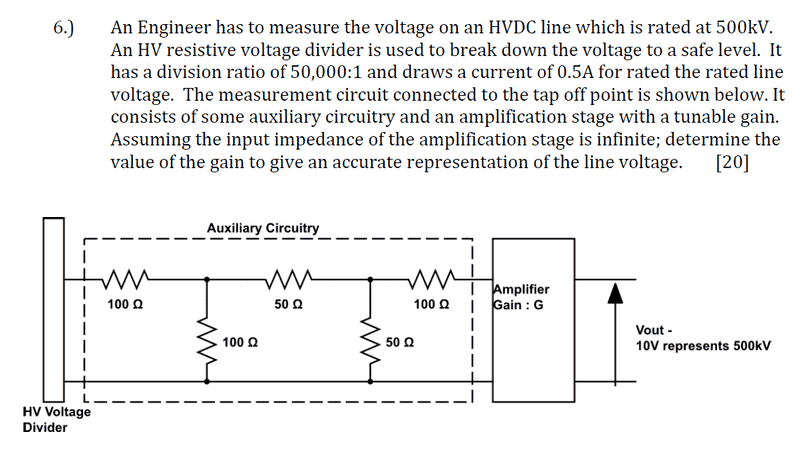# Gain Question

I have attachted the question as a picture:

my first attempt i calculated the voltage through the auxillary circuit to be 10V but i am confused of where the 0.5 A plays in this part, then i used nodal analysis to find the voltage input to the amplifier but up to that point i am confused of how to proceed, am i working the question through the right steps or am i missing somethingUltrafastPED
Gold Member
I think the voltage entering the circuit has a maximum of 10V from the voltage divider.

When I carry out the nodal analysis I get 1/3 of the starting voltage at the first node, and 1/6 of the starting voltage at the 2nd node ... and since the amplifier has "infinite impedance" it will see this 1/6 value.

Thus the gain must be 6 in order to recover the output of the voltage divider.

Clearly the 0.5A current is routed not through the amplifier, but down and around the return loop (implied ground). So the 0.5A is not part of the solution, but the source of the problem!

what i did is used the division ratio an the current and the high voltage to find 2 resistors that will give that division ratio but remember if u put the auxillary circuit over the resistor which gives that 10V it will change the resistance thus i found the the new voltage across it then found the voltage at the node going intot the amplifier, so remember what we want is 10 v across that resistor so the amplifier has to amplfiier it back to 10v, thats what i did